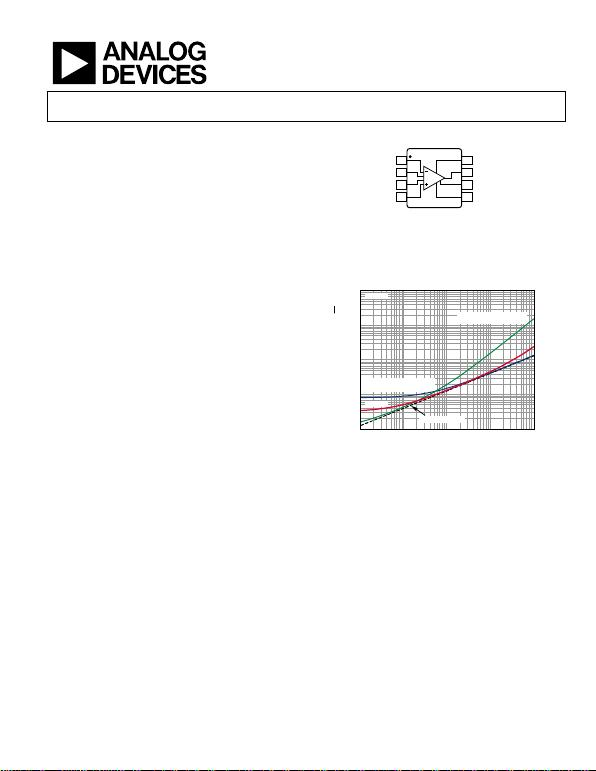3 nV/√Hz, Low Power
Instrumentation Amplifier
Rev. 0
Information furnished by Analog Devices is believed to be accurate and reliable. However, no
responsibility is assumed by Analog Devices for its use, nor for any infringements of patents or other
rights of third parties that may result from its use. Specifications subject to change without notice. No
license is granted by implication or otherwise under any patent or patent rights of Analog Devices.
One Technology Way, P.O. Box 9106, Norwood, MA 02062-9106, U.S.A.
Tel: 781.329.4700 www.analog.com
FEATURES
Low power
2.3 mA maximum supply current
Low noise
3.2 nV/√Hz maximum input voltage noise at 1 kHz
200 fA/√Hz current noise at 1 kHz
Excellent ac specifications
10 MHz bandwidth (G = 1)
2 MHz bandwidth (G = 100)
0.6 μs settling time to 0.001% (G = 10)
80 dB CMRR at 20 kHz (G = 1)
35 V/μs slew rate
94 dB CMRR minimum (G = 1)
0.2 μV/°C maximum input offset voltage drift
1 ppm/°C maximum gain drift (G = 1)
500 pA maximum input bias current
Inputs protected to 40 V from opposite supply
±2.5 V to ±18 V dual supply (5 V to 36 V single supply)
Gain set with a single resistor (G = 1 to 10,000)
APPLICATIONS
Medical instrumentation
Precision data acquisition
Microphone preamplification
Vibration analysis
Multiplexed input applications
PIN CONNECTION DIAGRAM
TOP VIEW
(Not to Scale)
–IN
1
R
G2
R
G3
+IN
4
+V
S
8
V
OUT
7
REF
6
–V
S
5
10123-001
Figure 1.
10µ
1n
100 1M
TOTAL NOISE DENSITY AT 1kHz (V/Hz)
SOURCE RESISTANCE, R
S
()
10123-078
100n
10n
1k 10k 100k
G = 100
BEST AVAILABLE
1mA LOW POWER IN-AMP
BEST AVAILABLE
7mA LOW NOISE IN-AMP
R
S
NOISE ONLY
Figure 2. Noise Density vs. Source Resistance
GENERAL DESCRIPTION
The AD8421 is a low cost, low power, extremely low noise, ultralow
bias current, high speed instrumentation amplifier that is ideally
suited for a broad spectrum of signal conditioning and data
acquisition applications. This product features extremely high
CMRR, allowing it to extract low level signals in the presence of
high frequency common-mode noise over a wide temperature
range.
The 10 MHz bandwidth, 35 V/µs slew rate, and 0.6 µs settling
time to 0.001% (G = 10) allow the AD8421 to amplify high speed
signals and excel in applications that require high channel count,
multiplexed systems. Even at higher gains, the current feedback
architecture maintains high performance; for example, at G = 100,
the bandwidth is 2 MHz and the settling time is 0.8 µs. The
AD8421 has excellent distortion performance, making it suitable
for use in demanding applications such as vibration analysis.
The AD8421 delivers 3 nV/√Hz input voltage noise and
200 fA/√Hz current noise with only 2 mA quiescent current,
making it an ideal choice for measuring low level signals. For
applications with high source impedance, the AD8421 employs
innovative process technology and design techniques to provide
noise performance that is limited only by the sensor.
The AD8421 uses unique protection methods to ensure robust
inputs while still maintaining very low noise. This protection
allows input voltages up to 40 V from the opposite supply rail
without damage to the part.
A single resistor sets the gain from 1 to 10,000. The reference
pin can be used to apply a precise offset to the output voltage.
The AD8421 is specified from −40°C to +85°C and has typical
performance curves to 125°C. It is available in 8-lead MSOP
and SOIC packages.Rev. 0 | Page 2 of 28
Features .............................................................................................. 1
Applications....................................................................................... 1
Pin Connection Diagram ................................................................ 1
General Description......................................................................... 1
Revision History ............................................................................... 2
Specifications..................................................................................... 3
Absolute Maximum Ratings............................................................ 8
Thermal Resistance...................................................................... 8
ESD Caution.................................................................................. 8
Pin Configuration and Function Descriptions............................. 9
Typical Performance Characteristics ........................................... 10
Theory of Operation ...................................................................... 20
Architecture................................................................................. 20
Gain Selection............................................................................. 20
Reference Terminal .................................................................... 21
Input Voltage Range................................................................... 21
Layout .......................................................................................... 21
Input Bias Current Return Path ............................................... 22
Input Voltages Beyond the Supply Rails.................................. 22
Calculating the Noise of the Input Stage................................. 23
Applications Information.............................................................. 25
Differential Output Configuration .......................................... 25
Outline Dimensions....................................................................... 27
Ordering Guide .......................................................................... 27
REVISION HISTORY
5/12—Revision 0: Initial Version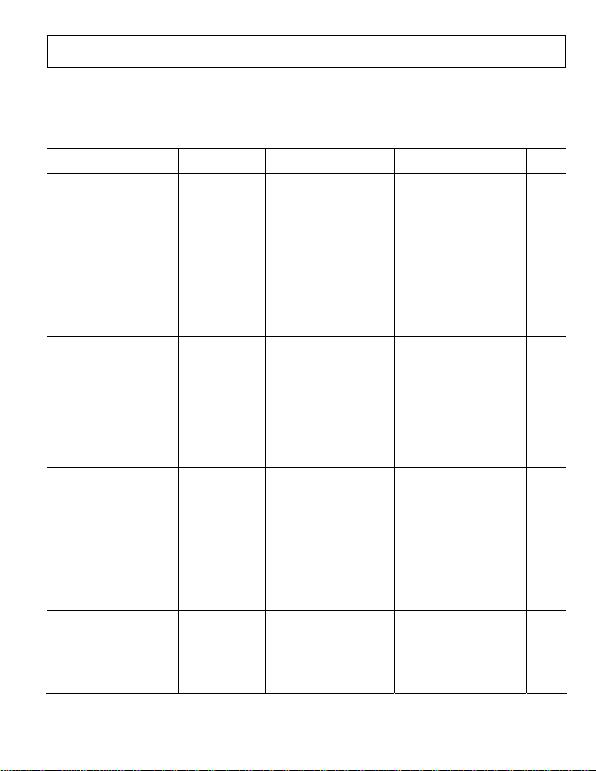Rev. 0 | Page 3 of 28
SPECIFICATIONS
VS = ±15 V, VREF = 0 V, TA = 25°C, G = 1, RL = 2 k, unless otherwise noted.
Table 1.
Parameter
Test Conditions/
Comments Min Typ Max Min Typ Max
Unit
COMMON-MODE REJECTION
RATIO (CMRR)
CMRR DC to 60 Hz with 1 kΩ
Source Imbalance
VCM = −10 V to +10 V
G = 1 86 94 dB
G = 10 106 114 dB
G = 100 126 134 dB
G = 1000 136 140 dB
Over Temperature, G = 1 T = −40°C to +85°C 80 93 dB
CMRR at 20 kHz VCM = −10 V to +10 V
G = 1 80 80 dB
G = 10 90 100 dB
G = 100 100 110 dB
G = 1000 110 120 dB
NOISE
Voltage Noise, 1 kHz1 V
IN+, VIN− = 0 V
Input Voltage Noise, eni 3 3.2 3 3.2 nV/√Hz
Output Voltage Noise, eno 60 60 nV/√Hz
Peak to Peak, RTI f = 0.1 Hz to 10 Hz
G = 1 2 2 2.2 μV p-p
G = 10 0.5 0.5 μV p-p
G = 100 to 1000 0.07 0.07 0.09 μV p-p
Current Noise
Spectral Density f = 1 kHz 200 200 fA/√Hz
Peak to Peak, RTI f = 0.1 Hz to 10 Hz 18 18 pA p-p
VOLTAGE OFFSET2
Input Offset Voltage, VOSI V
S = ±5 V to ±15 V 60 25 μV
Over Temperature TA = −40°C to +85°C 86 45 μV
Average TC 0.4 0.2 μV/°C
Output Offset Voltage, VOSO 350 250 μV
Over Temperature TA = −40°C to +85°C 0.66 0.45 mV
Average TC 6 5 μV/°C
Offset RTI vs. Supply (PSR) VS = ±2.5 V to ±18 V
G = 1 90 120 100 120 dB
G = 10 110 120 120 140 dB
G = 100 124 130 140 150 dB
G = 1000 130 140 140 150 dB
INPUT CURRENT
Input Bias Current 1 2 0.1 0.5 nA
Over Temperature TA = −40°C to +85°C 8 6 nA
Average TC 50 50 pA/°C
Input Offset Current 0.5 2 0.1 0.5 nA
Over Temperature TA = −40°C to +85°C 2.2 0.8 nA
Average TC 1 1 pA/°C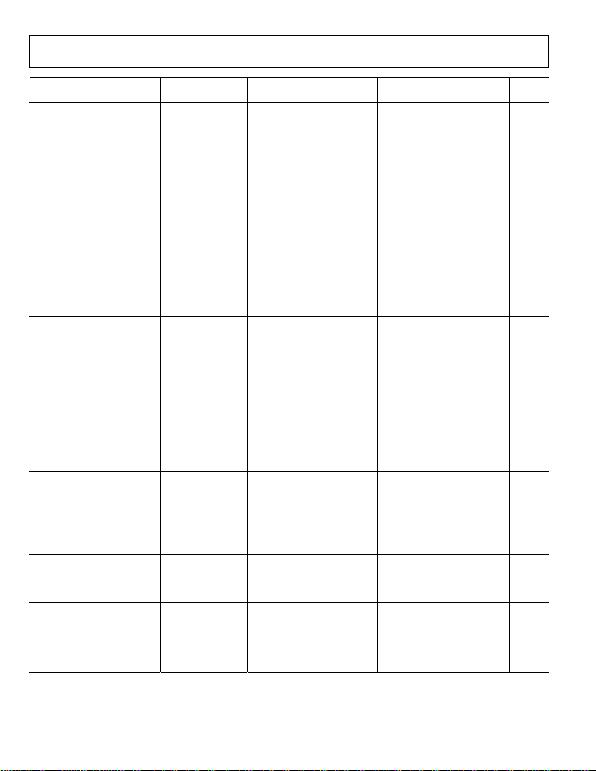Rev. 0 | Page 4 of 28
Parameter
Test Conditions/
Comments Min Typ Max Min Typ Max
Unit
DYNAMIC RESPONSE
Small Signal Bandwidth −3 dB
G = 1 10 10 MHz
G = 10 10 10 MHz
G = 100 2 2 MHz
G = 1000 0.2 0.2 MHz
Settling Time to 0.01% 10 V step
G = 1 0.7 0.7 μs
G = 10 0.4 0.4 μs
G = 100 0.6 0.6 μs
G = 1000 5 5 μs
Settling Time to 0.001% 10 V step
G = 1 1 1 μs
G = 10 0.6 0.6 μs
G = 100 0.8 0.8 μs
G = 1000 6 6 μs
Slew Rate
G = 1 to 100 35 35 V/μs
GAIN3 G = 1 + (9.9 kΩ/RG)
Gain Range 1 10,000 1 10,000 V/V
Gain Error VOUT = ±10 V
G = 1 0.02 0.01 %
G = 10 to 1000 0.2 0.1 %
Gain Nonlinearity VOUT = −10 V to +10 V
G = 1 RL ≥ 2 kΩ 1 1 ppm
R
L = 600 Ω 1 3 1 3 ppm
G = 10 to 1000 RL ≥ 600 Ω 30 50 30 50 ppm
V
OUT = −5 V to +5 V 5 10 5 10 ppm
Gain vs. Temperature3
G = 1 5 0.1 1 ppmC
G > 1 −50 −50 ppm/°C
INPUT
Input Impedance
Differential 30||3 30||3 GΩ||pF
Common Mode 30||3 30||3 GΩ||pF
Input Operating Voltage Range4 V
S = ±2.5 V to ±18 V −VS + 2.3 +VS − 1.8 −VS + 2.3 +VS − 1.8 V
Over Temperature TA = −40°C −VS + 2.5 +VS − 2.0 −VS + 2.5 +VS − 2.0 V
T
A = +85°C VS + 2.1 +VS − 1.8 −VS + 2.1 +VS − 1.8 V
OUTPUT RL = 2 kΩ
Output Swing VS = ±2.5 V to ±18 V −VS + 1.2 +Vs − 1.6 −VS + 1.2 +VS − 1.6 V
Over Temperature TA = −40°C to +85°C −VS + 1.2 +Vs − 1.6 −VS + 1.2 +VS − 1.6 V
Short-Circuit Current 65 65 mA
REFERENCE INPUT
RIN 20 20
IIN V
IN+, VIN− = 0 V 20 24 20 24 μA
Voltage Range −VS +VS −VS +VS V
Reference Gain to Output 1 ±
0.0001
1 ±
0.0001
V/V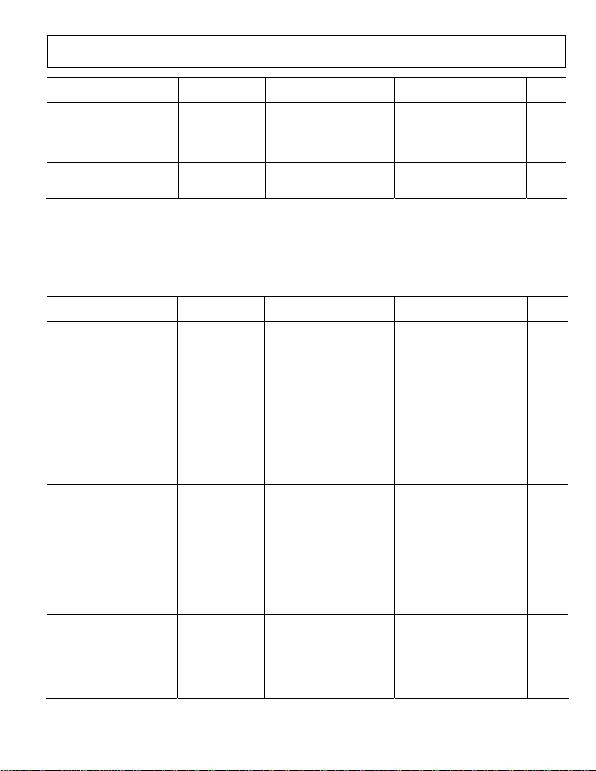Rev. 0 | Page 5 of 28
Parameter
Test Conditions/
Comments Min Typ Max Min Typ Max
Unit
POWER SUPPLY
Operating Range Dual supply ±2.5 ±18 ±2.5 ±18 V
Single supply 5 36 5 36 V
Quiescent Current 2 2.3 2 2.3 mA
Over Temperature TA = −40°C to +85°C 2.6 2.6 mA
TEMPERATURE RANGE
For Specified Performance −40 +85 −40 +85 °C
Operational5 −40 +125 −40 +125 °C
1 Total voltage noise = √(eni2 + (eno/G)2 + eRG2). See the Th section for more information. eory of Operation
2 Total RTI VOS = (VOSI) + (VOSO/G).
3 These specifications do not include the tolerance of the external gain setting resistor, RG. For G > 1, add RG errors to the specifications given in this table.
4 Input voltage range of the AD8421 input stage only. The input range can depend on the common-mode voltage, differential voltage, gain, and reference voltage.
See the section for more details. Input Voltage Range
5 See the section for expected operation between 85°C and 125°C. Typical Performance Characteristics
Table 2.
Parameter
Test Conditions/
Comments Min Typ Max Min Typ Max
Unit
COMMON-MODE REJECTION
RATIO (CMRR)
CMRR DC to 60 Hz with 1 kΩ
Source Imbalance
VCM = −10 V to +10 V
G = 1 84 92 dB
G = 10 104 112 dB
G = 100 124 132 dB
G = 1000 134 140 dB
Over Temperature, G = 1 TA = −40°C to +85°C 80 90 dB
CMRR at 20 kHz VCM = −10 V to +10 V
G = 1 80 80 dB
G = 10 90 90 dB
G = 100 100 100 dB
G = 1000 100 100 dB
NOISE
Voltage Noise, 1 kHz1 V
IN+, VIN− = 0 V
Input Voltage Noise, eni 3 3.2 3 3.2 nV/√Hz
Output Voltage Noise, eno 60 60 nV/√Hz
Peak to Peak, RTI f = 0.1 Hz to 10 Hz
G = 1 2 2 2.2 μV p-p
G = 10 0.5 0.5 μV p-p
G = 100 to 1000 0.07 0.07 0.09 μV p-p
Current Noise
Spectral Density f = 1 kHz 200 200 fA/√Hz
Peak to Peak, RTI f = 0.1 Hz to 10 Hz 18 18 pA p-p
VOLTAGE OFFSET2
Input Offset Voltage, VOSI V
S = ±5 V to ±15 V 70 50 μV
Over Temperature TA = −40°C to +85°C 135 135 μV
Average TC 0.9 0.9 μV/°C
Output Offset Voltage, VOSO 600 400 μV
Over Temperature TA = −40°C to +85°C 1 1 mV
Average TC 9 9 μV/°CRev. 0 | Page 6 of 28
Parameter
Test Conditions/
Comments Min Typ Max Min Typ Max
Unit
Offset RTI vs. Supply (PSR) VS = ±2.5 V to ±18 V
G = 1 90 120 100 120 dB
G = 10 110 120 120 140 dB
G = 100 124 130 140 150 dB
G = 1000 130 140 140 150 dB
INPUT CURRENT
Input Bias Current 1 2 0.1 1 nA
Over Temperature TA = −40°C to +85°C 8 6 nA
Average TC 50 50 pA/°C
Input Offset Current 0.5 2 0.1 1 nA
Over Temperature TA = −40°C to +85°C 3 1.5 nA
Average TC 1 1 pA/°C
DYNAMIC RESPONSE
Small Signal Bandwidth −3 dB
G = 1 10 10 MHz
G = 10 10 10 MHz
G = 100 2 2 MHz
G = 1000 0.2 0.2 MHz
Settling Time 0.01% 10 V step
G = 1 0.7 0.7 μs
G = 10 0.4 0.4 μs
G = 100 0.6 0.6 μs
G = 1000 5 5 μs
Settling Time 0.001% 10 V step
G = 1 1 1 μs
G = 10 0.6 0.6 μs
G = 100 0.8 0.8 μs
G = 1000 6 6 μs
Slew Rate
G = 1 to 100 35 35 V/μs
GAIN3 G = 1 + (9.9 kΩ/RG)
Gain Range 1 10,000 1 10,000 V/V
Gain Error VOUT = ±10 V
G = 1 0.05 0.02 %
G = 10 to 1000 0.3 0.2 %
Gain Nonlinearity VOUT = −10 V to +10 V
G = 1 RL ≥ 2 kΩ 1 1 ppm
R
L = 600 Ω 1 3 1 3 ppm
G = 10 to 1000 RL ≥ 600 Ω 30 50 30 50 ppm
V
OUT = −5 V to +5 V 5 10 5 10 ppm
Gain vs. Temperature3
G = 1 5 0.1 1 ppm/°C
G > 1 −50 −50 ppm/°C
INPUT
Input Impedance
Differential 30||3 30||3 GΩ||pF
Common Mode 30||3 30||3 GΩ||pF
Input Operating Voltage
Range4
VS = ±2.5 V to ±18 V −VS + 2.3 +VS − 1.8 −VS + 2.3 +VS − 1.8 V
Over Temperature TA = −40°C −VS + 2.5 +VS − 2.0 VS + 2.5 +VS − 2.0 V
T
A = +85°C −VS + 2.1 +VS − 1.8 −VS + 2.1 +VS − 1.8 VRev. 0 | Page 7 of 28
Parameter
Test Conditions/
Comments Min Typ Max Min Typ Max
Unit
OUTPUT RL = 2 kΩ
Output Swing VS = ±2.5 V to ±18 V −VS + 1.2 +VS 1.6 −VS + 1.2 +Vs − 1.6 V
Over Temperature TA = −40°C to +85°C −VS + 1.2 +VS − 1.6 −VS + 1.2 +Vs − 1.6 V
Short-Circuit Current 65 65 mA
REFERENCE INPUT
RIN 20 20
IIN V
IN+, VIN− = 0 V 20 24 20 24 μA
Voltage Range −VS +VS −VS +VS V
Reference Gain to Output 1 ±
0.0001
1 ±
0.0001
V/V
POWER SUPPLY
Operating Range Dual supply ±2.5 ±18 ±2.5 ±18 V
Single supply 5 36 5 36 V
Quiescent Current 2 2.3 2 2.3 mA
Over Temperature TA = −40°C to +85°C 2.6 2.6 mA
TEMPERATURE RANGE
For Specified Performance −40 +85 −40 +85 °C
Operational5 −40 +125 −40 +125 °C
1 Total voltage noise = √(eni2 + (eno/G)2 + eRG2). See the Th section for more information. eory of Operation
2 Total RTI VOS = (VOSI) + (VOSO/G).
3 These specifications do not include the tolerance of the external gain setting resistor, RG. For G > 1, add RG errors to the specifications given in this table.
4 Input voltage range of the AD8421 input stage only. The input range can depend on the common-mode voltage, differential voltage, gain, and reference voltage.
5 See the section for expected operation between 85°C and 125°C. Typical Performance Characteristics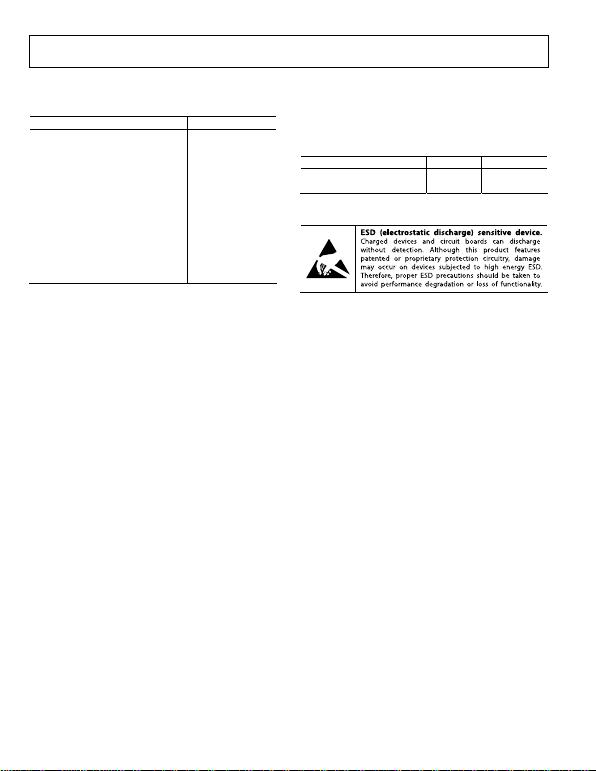Rev. 0 | Page 8 of 28
ABSOLUTE MAXIMUM RATINGS
Table 3.
Parameter Rating
Supply Voltage ±18 V
Output Short-Circuit Current Duration Indefinite
Maximum Voltage at −IN or +IN1 −VS + 40 V
Minimum Voltage at −IN or +IN +VS − 40 V
Maximum Voltage at REF2 +VS + 0.3 V
Minimum Voltage at REF −VS − 0.3 V
Storage Temperature Range −65°C to +150°C
Operating Temperature Range −40°C to +125°C
Maximum Junction Temperature 150°C
ESD
Human Body Model 2 kV
Charged Device Model 1.25 kV
Machine Model 0.2 kV
1 For voltages beyond these limits, use input protection resistors. See the
2 There are ESD protection diodes from the reference input to each supply, so
REF cannot be driven beyond the supplies in the same way that +IN and −IN
Stresses above those listed under Absolute Maximum Ratings
may cause permanent damage to the device. This is a stress
rating only; functional operation of the device at these or any
other conditions above those indicated in the operational
section of this specification is not implied. Exposure to absolute
maximum rating conditions for extended periods may affect
device reliability.
THERMAL RESISTANCE
θJA is specified for a device in free air using a 4-layer JEDEC
printed circuit board (PCB).
Table 4.
Package θJA Unit
ESD CAUTION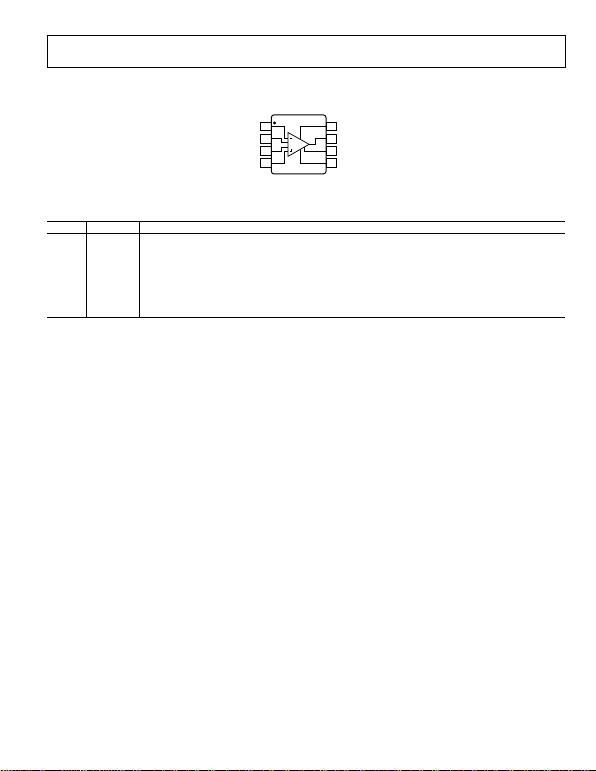Rev. 0 | Page 9 of 28
PIN CONFIGURATION AND FUNCTION DESCRIPTIONS
TOP VIEW
(Not to Scale)
–IN
1
R
G2
R
G3
+IN
4
+V
S
8
V
OUT
7
REF
6
–V
S
5
10123-002
Figure 3. Pin Configuration
Table 5. Pin Function Descriptions
Pin No. Mnemonic Description
1 −IN Negative Input Terminal.
2, 3 RG Gain Setting Terminals. Place resistor across the RG pins to set the gain. G = 1 + (9.9 kΩ/RG).
4 +IN Positive Input Terminal.
5 −VS Negative Power Supply Terminal.
6 REF Reference Voltage Terminal. Drive this terminal with a low impedance voltage source to level shift the output.
7 VOUT Output Terminal.
8 +VS Positive Power Supply Terminal.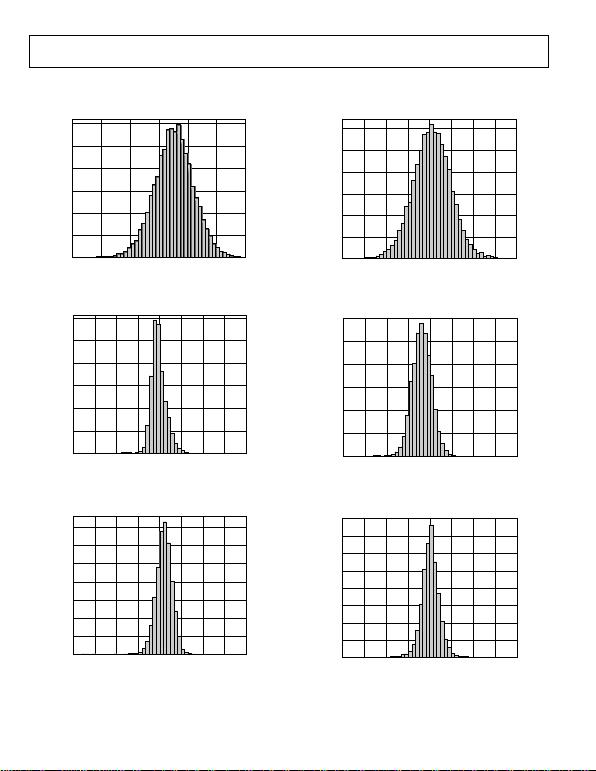Rev. 0 | Page 10 of 28
TYPICAL PERFORMANCE CHARACTERISTICS
TA = 25°C, VS = ±15 V, VREF = 0 V, RL = 2 kΩ, unless otherwise noted.
600
0
100
200
300
400
500
–60 –40 –20 20 4006
UNITS
INPUT OFFSET VOLTAGE (µV)
0
10123-003
Figure 4. Typical Distribution of Input Offset Voltage
1800
0
300
600
900
1200
1500
–2.0 –1.5 1.51.0–1.0 –0.5 0 0.5 2.0
UNITS
INPUT BIAS CURRENT (nA)
10123-004
Figure 5. Typical Distribution of Input Bias Current
1400
0
200
400
600
800
1200
1000
–20 –15 1510–10 –5 0 5 20
UNITS
PSRR (µV/V)
10123-005
Figure 6. Typical Distribution of PSRR (G = 1)
600
0
100
200
300
400
500
–400 –300 300200–200 –100 0 100 400
UNITS
OUTPUT OFFSET VOLTAGE (µV)
10123-006
Figure 7. Typical Distribution of Output Offset Voltage
800
1000
1200
600
0
200
400
–2.0 –1.5 –1.0 –0.5 0.5 1.0 1.502
UNITS
INPUT OFFSET CURRENT (nA)
10123-007
.0
Figure 8. Typical Distribution of Input Offset Current
600
800
1000
1200
1400
1600
0
200
400
–120 1209060300–30–60–90
UNITS
CMRR (µV/V)
10123-008
Figure 9. Typical Distribution of CMRR (G = 1)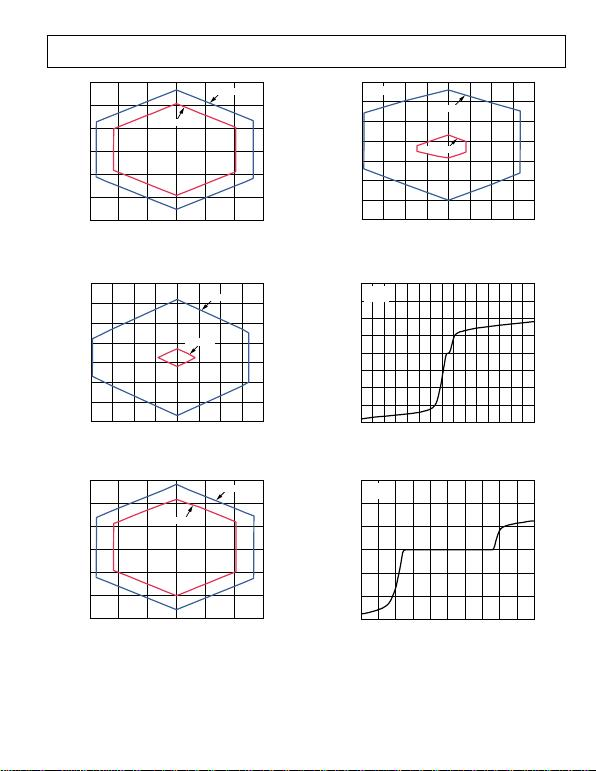Rev. 0 | Page 11 of 28
15
10
5
0
–15
–10
–5
–15 15105–5–10 0
COMMON-MODE VOLTAGE (V)
OUTPUT VOLTAGE (V)
V
S
= ±15V
V
S
= ±12V
10123-009
G = 1
Figure 10. Input Common-Mode Voltage vs. Output Voltage;
VS = ±12 V and ±15 V (G = 1)
4
3
2
0
1
–3
–2
–1
–4 4321–3 –2 –1 0
COMMON-MODE VOLTAGE (V)
OUTPUT VOLTAGE (V)
V
S
= ±5V
V
S
= ±2.5V
10123-010
G = 1
Figure 11. Input Common-Mode Voltage vs. Output Voltage;
VS = ±2.5 V and ±5 V (G = 1)
15
10
5
0
–15
–10
–5
–15 15105–5–10 0
COMMON-MODE VOLTAGE (V)
OUTPUT VOLTAGE (V)
V
S
= ±15V
V
S
= ±12V
10123-011
G = 100
Figure 12. Input Common-Mode Voltage vs. Output Voltage;
VS = ±12 V and ±15 V (G = 100)
4
3
2
0
1
–3
–2
–1
–4 4321–3 –2 –1 0
COMMON-MODE VOLTAGE (V)
OUTPUT VOLTAGE (V)
V
S
= ±5V
V
S
= ±2.5V
10123-012
G = 100
Figure 13. Input Common-Mode Voltage vs. Output Voltage;
VS = ±2.5 V and ±5 V (G = 100)
40
–40
–35 40
INPUT CURRENT (mA)
INPUT VOLTAGE (V)
–30
–20
–10
0
10
20
30
30252015105 0 5 101520253035
V
S
= 5V
G = 1
10123-013
Figure 14. Input Overvoltage Performance; G = 1, +VS = 5 V, −VS = 0 V
30
–30
–25 25
INPUT CURRENT (mA)
INPUT VOLTAGE (V)
–20
–10
0
10
20
–20 –15 –10 –5 0 5 10 15 20
V
S
= ±15V
G = 1
10123-014
Figure 15. Input Overvoltage Performance; G = 1, VS = ±15 V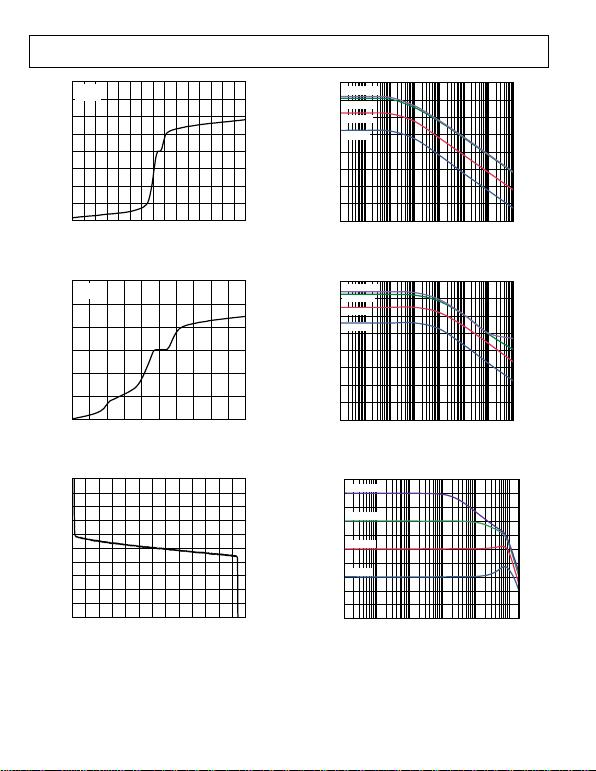Rev. 0 | Page 12 of 28
40
–40
–35 40
INPUT CURRENT (mA)
INPUT VOLTAGE (V)
–30
–20
–10
0
10
20
30
30252015105 0 5 101520253035
V
S
= 5V
G = 100
10123-015
Figure 16. Input Overvoltage Performance; +VS = 5 V, −VS = 0 V, G = 100
30
–30
–25 25
INPUT CURRENT (mA)
INPUT VOLTAGE (V)
–20
–10
0
10
20
–20 –15 –10 –5 0 5 10 15 20
V
S
= ±15V
G = 100
10123-016
Figure 17. Input Overvoltage Performance; VS = ±15 V, G = 100
2.5
–2.5
–12 14
BIAS CURRENT (nA)
COMMON-MODE VOLTAGE (V)
–2.0
–1.5
–1.0
–0.5
0
0.5
1.0
1.5
2.0
108642024681012
10123-017
Figure 18. Input Bias Current vs. Common-Mode Voltage
160
140
120
100
80
60
40
20
0
0.1 1 10 100 1k 10k 100k 1M
FREQUENCY (Hz)
POSITIVE PSRR (dB)
GAIN = 100
GAIN = 10
GAIN = 1
GAIN = 1000
10123-018
Figure 19. Positive PSRR vs. Frequency
160
140
120
100
80
60
40
20
0
0.1 1 10 100 1k 10k 100k 1M
FREQUENCY (Hz)
NEGATIVE PSRR (dB)
GAIN = 100
GAIN = 10
GAIN = 1
GAIN = 1000
10123-019
Figure 20. Negative PSRR vs. Frequency
70
–30
100 1k 10k 100k 1M 10M
GAIN (dB)
FREQUENCY (Hz)
–20
–10
0
10
20
30
40
50
60
GAIN = 100
GAIN = 10
GAIN = 1
GAIN = 1000
10123-020
Figure 21. Gain vs. Frequency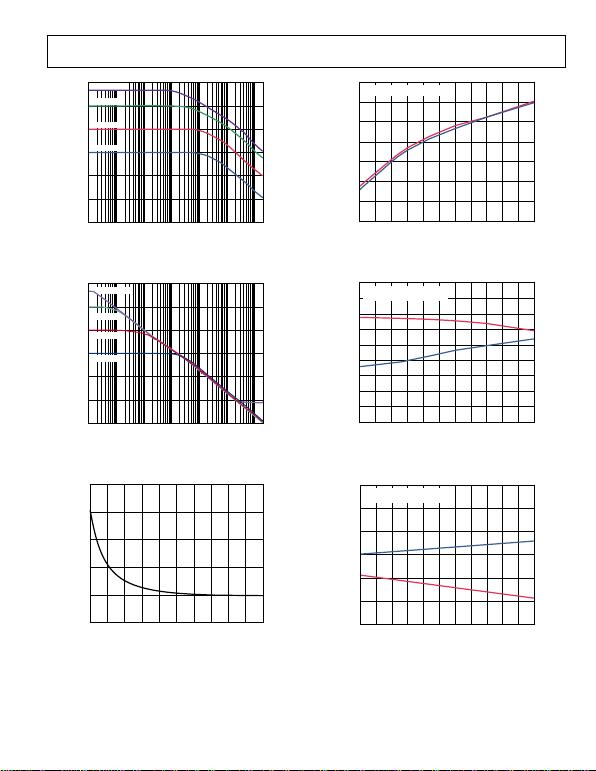Rev. 0 | Page 13 of 28
40
60
80
100
CMRR (dB)
120
140
160
0.1 1 10 100 1k 10k 100k
FREQUENCY (Hz)
GAIN = 1000
GAIN = 100
GAIN = 10
GAIN = 1
10123-021
Figure 22. CMRR vs. Frequency
40
60
80
100
CMRR (dB)
120
140
160
0.1 1 10 100 1k 10k 100k
FREQUENCY (Hz)
GAIN = 1000
GAIN = 100
GAIN = 10
GAIN = 1
10123-022
Figure 23. CMRR vs. Frequency, 1 kΩ Source Imbalance
2.0
1.5
0.5
0
1.0
–0.5
05
CHANGE IN INPUT OFFSET VOLTAGE (µV)
WARM-UP TIME (Seconds)
5 1015202530354045 0
10123-023
Figure 24. Change in Input Offset Voltage (VOSI) vs. Warm-Up Time
6
–8
–40 125
BIAS CURRENT (nA)
TEMPERATURE (°C)
–6
–4
–2
0
2
4
25105 203550658095110
REPRESENTATIVE SAMPLES
10123-024
Figure 25. Input Bias Current vs. Temperature
100
–80
–40 125
GAIN ERROR (µV/V)
TEMPERATURE (°C)
–60
–40
–20
0
20
40
60
80
25105 203550658095110
REPRESENTATIVE SAMPLES
GAIN = 1
10123-025
Figure 26. Gain vs. Temperature (G = 1)
15
10
5
–15
–10
–5
0
–40 125
CMRR (µV/V)
TEMPERATURE (°C)
25105 203550658095110
REPRESENTATIVE SAMPLES
GAIN = 1
10123-074
Figure 27. CMRR vs. Temperature (G = 1)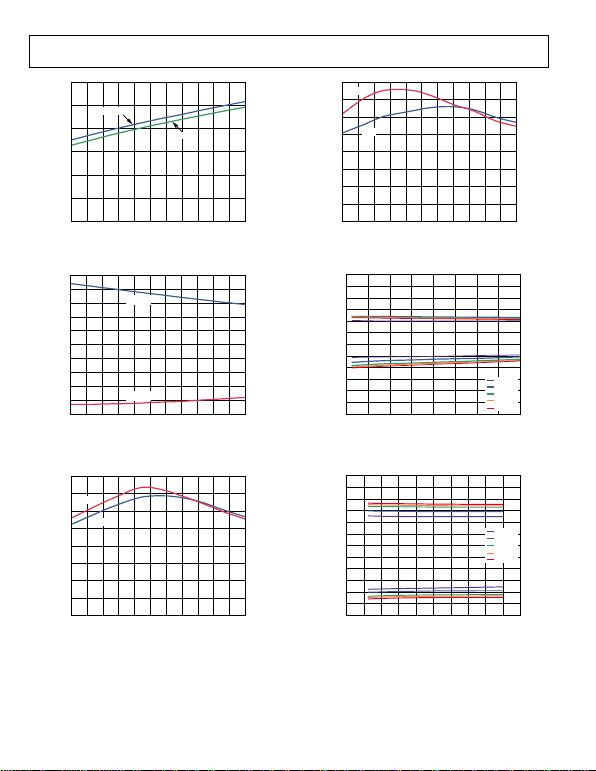Rev. 0 | Page 14 of 28
3.0
2.5
2.0
1.5
1.0
0.5
0
–40 125
SUPPLY CURRENT (mA)
TEMPERATURE (°C)
–25 –10 5 20 35 50 65 80 95 110
V
S
= ±15V
V
S
= ±5V
10123-026
Figure 28. Supply Current vs. Temperature (G = 1)
80
–120
–40 110
SHORT-CIRCUIT CURRENT (mA)
TEMPERATURE (°C)
–100
–80
–60
–40
–20
0
20
40
60
–25 –10 5 20 35 50 65 80 95 125
I
SHORT+
I
SHORT–
10123-027
Figure 29. Short-Circuit Current vs. Temperature (G = 1)
40
0
–40 125
SLEW RATE (V/µs)
TEMPERATURE (°C)
5
10
15
20
25
30
35
–25 –10 5 20 35 50 65 80 95 110
–SR
+SR
10123-028
Figure 30. Slew Rate vs. Temperature, VS = ±15 V (G = 1)
40
0
–40 125
SLEW RATE (V/µs)
TEMPERATURE (°C)
5
10
15
20
25
30
35
–25 –10 5 20 35 50 65 80 95 110
–SR
+SR
10123-029
Figure 31. Slew Rate vs. Temperature, VS = ±5 V (G = 1)
+
V
S
–V
S
21
INPUT VOLTAGE (V)
REFERRED TO SUPPLY VOLTAGES
SUPPLY VOLTAGE (±V
S
)
4 6 8 10121416
–0.5
–1.0
–1.5
–2.0
–2.5
+0.5
+1.0
+1.5
+2.0
+2.5
8
–40°C
+25°C
+85°C
+105°C
+125°C
10123-030
Figure 32. Input Voltage Limit vs. Supply Voltage
+
V
S
–V
S
02 2018
OUTPUT VOLTAGE (V)
REFERRED TO SUPPLY VOLTAGES
SUPPLY VOLTAGE (±V
S
)
4 6 8 10 12 14 16
–0.5
–1.0
–1.5
–2.0
–2.5
+0.5
+1.0
+1.5
+2.0
+2.5
–40°C
+25°C
+85°C
+105°C
+125°C
10123-031
Figure 33. Output Voltage Swing vs. Supply Voltage, RL = 10 kΩ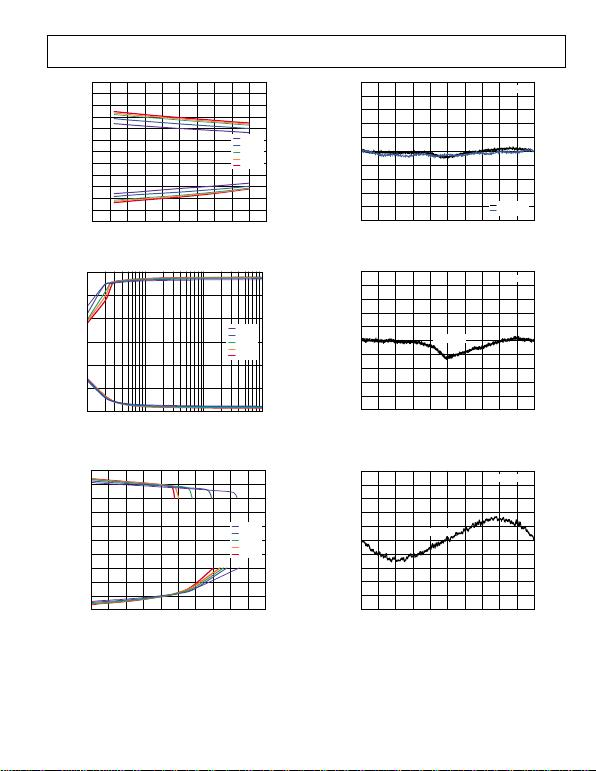Rev. 0 | Page 15 of 28
+
V
S
–V
S
02 2018
OUTPUT VOLTAGE (V)
REFERRED TO SUPPLY VOLTAGES
SUPPLY VOLTAGE (±V
S
)
4 6 8 10 12 14 16
–0.5
–1.0
–1.5
–2.0
–2.5
+0.5
+1.0
+1.5
+2.0
+2.5
–40°C
+25°C
+85°C
+105°C
+125°C
10123-032
Figure 34. Output Voltage Swing vs. Supply Voltage, RL = 600 Ω
15
10
–15
–10
–5
5
0
100 100k
OUTPUT VOLTAGE SWING (V)
1k 10k
–40°C
+25°C
+85°C
+105°C
+125°C
10123-033
Figure 35. Output Voltage Swing vs. Load Resistance
+
V
S
–VS0 0.01 0.100.09
OUTPUT VOLTAGE SWING (V)
REFERRED TO SUPPLY VOLTAGES
OUTPUT CURRENT (A)
0.02 0.03 0.04 0.05 0.06 0.07 0.08
–2
–4
–6
–8
+2
+4
+6
+8
–40°C
+25°C
+85°C
+105°C
+125°C
10123-034
Figure 36. Output Voltage Swing vs. Output Current
5
4
3
–5
–4
–3
–2
–1
0
1
2
–10 –8 –6 –4 –2 0 2 4 6 8 10
NONLINEARITY (ppm)
OUTPUT VOLTAGE (V)
GAIN = 1
R
L
= 2k
R
L
= 10k
10123-035
Figure 37. Gain Nonlinearity (G = 1), RL = 10 kΩ, 2 kΩ
5
4
3
–5
–4
–3
–2
–1
0
1
2
–10 –8 –6 –4 –2 0 2 4 6 8 10
NONLINEARITY (ppm)
OUTPUT VOLTAGE (V)
GAIN = 1
R
L
= 600
10123-036
Figure 38. Gain Nonlinearity (G = 1), RL = 600 Ω
100
80
60
–100
–80
–60
–40
–20
0
20
40
–10 –8 –6 –4 –2 0 2 4 6 8 10
NONLINEARITY (ppm)
OUTPUT VOLTAGE (V)
GAIN = 1000
R
L
= 600
10123-072
Figure 39. Gain Nonlinearity (G = 1000), RL = 600 Ω, VOUT = ±10 V